﻿ Tip Deflection Control for a Rotating Flexible Link

Tip Deflection Control for a Rotating Flexible Link

Somer M. Nacy, Osamah F. Fakhri, Rafal M. Khaleel

American Journal of Mechanical Engineering

Tip Deflection Control for a Rotating Flexible Link

Somer M. Nacy1,, Osamah F. Fakhri1, Rafal M. Khaleel2

Abstract

The tip deflection control for a rotating flexible link is investigated according to a novel approach. This approach considers the variation of the moment of inertia of the flexible link during rotation, thus reducing the tip deflection due to the generated inertia force. The link mechanism implemented in this study was made of Perspex and it consists of two parts along its length, one is fixed and the other is rotating about an axis normal to the direction of the rotation of the link mechanism. An MMA7631 accelerometer + gyro was used to measure the tip deflection, while the control process was achieved by using MEGA Arduino board. Tests were carried out for three cases of end loads, namely, 0, 27.5, 59.5 gm. Results show that the application of the proposed control system succeeded in reducing the tip deflection as given by the desired value, which was chosen to be 50% and 80% for all the cases of end loads.

• Somer M. Nacy, Osamah F. Fakhri, Rafal M. Khaleel. Tip Deflection Control for a Rotating Flexible Link. American Journal of Mechanical Engineering. Vol. 4, No. 4, 2016, pp 163-168. http://pubs.sciepub.com/ajme/4/4/5
• Nacy, Somer M., Osamah F. Fakhri, and Rafal M. Khaleel. "Tip Deflection Control for a Rotating Flexible Link." American Journal of Mechanical Engineering 4.4 (2016): 163-168.
• Nacy, S. M. , Fakhri, O. F. , & Khaleel, R. M. (2016). Tip Deflection Control for a Rotating Flexible Link. American Journal of Mechanical Engineering, 4(4), 163-168.
• Nacy, Somer M., Osamah F. Fakhri, and Rafal M. Khaleel. "Tip Deflection Control for a Rotating Flexible Link." American Journal of Mechanical Engineering 4, no. 4 (2016): 163-168.

 Import into BibTeX Import into EndNote Import into RefMan Import into RefWorks

1234
Prev Next

1. Introduction

Flexible link manipulators exhibit one main advantage of being light in weight as compared to rigid link manipulators. Hence, they can achieve high speed operation with lower energy consumption. Due to the flexible nature of the system it is difficult to achieve accuracy in positioning of the tip. Many industrial flexible manipulators face the problem of vibration during high speed motion, for which the operation with high precision is severely limited. These conflicting requirements between high speed and high accuracy have rendered the robotic assembly task to be a challenging research.

Research work on the control of flexible manipulators was initiated in the 80’s of the last century. After then many researches have been achieved as related to the control of the tip deflection.

Different approaches and strategies were used to control the tip deflection of flexible manipulator, such as, nested feedback lops , sliding – mode and shape- input concept , integral manifolds and input – output decoupling , passivity based controller and observer based controller , linear quadratic regulator (LQR) , passive velocity feedback and strain feedback approaches , time domain approach and frequency domain H-Infinity approaches , modified PID (MPID) control , and nonlinear adaptive controller .

It is well known that to attain the control goals for a system over a period of time, the controller has to cope with significant uncertainty for which fixed feedback robust controllers or adaptive controllers cannot deal with . Hence intelligent control methods were adopted. Under these considerations, intelligent controllers were used to control the tip deflection of the flexible manipulator through different methods, such as fuzzy logic [11, 12, 13, 14], Neural network [15, 16, 17], and genetic algorithm [18, 19]. Recently, piezoelectric materials in the form of layers or self-acting actuators are used to control the vibration of flexible structures [20, 21, 22, 23, 24].

In this work, a special purpose single link flexible manipulator is fabricated and facilitated with all the required instruments, hence to achieve an active closed loop control system for the link tip deflection. The implementation of this system was based upon the variation of the second moment of area of the flexible link in a trial to control the lateral stiffness of the link. As compared to other research work, the need for complicated electronics and software is minimized to attain the target, also an experimental verification has been achieved to validate the proposed approach.

2. Theoretical Evidence

The dynamic equations are derived using Lagrange’s energy equations in term of a set of generalized variables α and θ, as follows,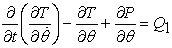(1)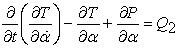(2)

Where, T is the total kinetic energy, P is the total potential energy, Qi is the ith generalized force within the ith degree of freedom,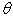and α, as shown in Figure 1, are the angles of base rotation and tip deflection respectively.Download asVeiw figureFigures index
Figure 1. Single link flexible manipulator

The total kinetic energy is,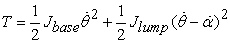(3)

Where, Jbase is the mass moment of inertia of the base, Jlump is the combined mass moment of inertia of the link and the attached payload, which is found to be as,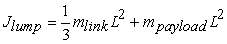(4)

Where m link and m payload are the masses of the link and the payload respectively, L is he length of the beam.

The total potential energy is,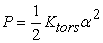(5)

Where K tors is the torsional stiffness of the flexible link about the axis of rotation of the base, which is related to lateral stiffness as follows,(6)

In turn the lateral stiffness of the flexible link can be found to be as,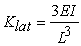(7)

Where, E is the modules of elasticity of the flexible beam and I is the second moment of area of the cross – section of the flexible link.

Substituting the total kinetic energy and the total potential energy in Lagrange’s equations to obtain the dynamic equations for the system, which can be presented in a state space form as follows,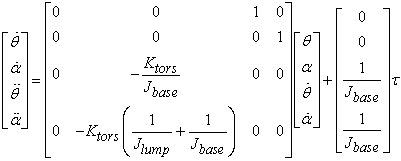(8)

Where τ is the torque applied to the rotational base.

It is clear, from eq.(8) that one of the dominating factors affecting the dynamic performance is the torsional stiffness of the flexible link, which in turn is dependent on the second moment of area of the link cross-section. Referring to eq. (6) and (7), the torsional stiffness increases as the second moment of area increases. The mechanism adopted in this work, hence to increase the second moment of area, is clarified in Figure 2,Download asVeiw figureFigures index
Figure 2. Cross-section of flexible link

The second moment of area of the link about the X-X axis is,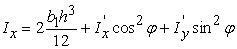(9)

where,(10)

From the above, it is clear that as the angle ϕ increases the second moment of area increases, reaching its maximum value at ϕ = 90˚. Also, as the ratio b2/(2d1+b2) increases, the variation of Ix with ϕ will be increased, thus obtaining higher stiffness in the lateral direction.

3. Experimentation

The flexible link manipulator applied in this work is presented pictorially in Figure 3 and schematically as shown in Figure 4.Download asVeiw figureFigures index
Figure 3. Single link flexible manipulatorDownload asVeiw figureFigures index
Figure 4. Schematic of the flexible link manipulator

It consists of the flexible link mechanism, MMA7361 accelerometer and Arduino Mega 2560 board. The flexible link, of mass 76 gm, is fixed on a Teflon hub which rotates about the B-B axis by the base servo motor, the moment of inertia of this hub is 0.229 kg-m2. The link rotation about the A-A axis, normal to the hub rotation, is controlled by the link servo motor which is fixed inside the Teflon hub as shown in Figure 4. An detailed view for the fixed part and rotating part of the flexible beam, as fixed to the shaft of the link servomotor, is depicted in Figure 5.Download asVeiw figureFigures index
Figure 5. Fixed and rotating parts of the flexible beam

The cross-sectional view of the flexible beam, shown in Figure 2 has the following dimensions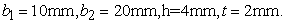The specification of the base servo motor and the link servo motor are presented in Table 1 and Table 2 respectively.

Table 1. specification of base servomotor

A pay load is attached to the free end of the link, at which the deflection is measured by means of the accelerometer. A desired speed of 8 rad/s and desired angle of rotation of 90o is fed to the Arduino, hence to drive the base servomotor. An allowable tip deflection is fed to the control system. This deflection is considered as a percentage of the maximum deflection occurred at the tip for a specified tip load in case where the control system is not activated. In this investigation, two allowable percentages were considered, namely, 50% and 80%. The induced tip deflection measured by the accelerometer is fed back and compared to the allowable deflection through the Arduino board, which in turn activates the angle of rotation of the link servomotor, thus rotating the inner part of the link to increase the second moment of area, hence increasing the lateral link stiffness and decreasing the tip deflection. The angle of rotation of the link servomotor is limited by the difference between the measured tip deflection and the allowable tip deflection. A block diagram of the control system is shown in Figure 6.Download asVeiw figureFigures index
Figure 6. Block diagram of the control system

The equation governing the rotation of the base servomotor is,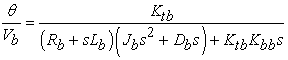(11)

where,

Vb: voltage of the base servomotor

Rb: resistance of the base servomotor

Lb: inductance of the base servomotor

Db: damping coefficient of the base servomotor

Ktb: torque constant of the base servomotor

Kbb: back emf constant of the base servomotor

Jb: moment of inertia of the mass connected to the shaft of the base servomotor, Jb = Jbase + Jlump

The link servomotor is governed by,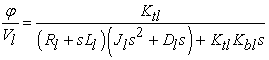(12)

where,

Vl: voltage of the link servomotor

Rl: resistance of the link servomotor

Ll: inductance of the link servomotor

Dl: damping coefficient of the link servomotor

Ktl: torque constant of the link servomotor

Kbl: back emf constant of the link servomotor

Jl: moment of inertia of the mass connected to the shaft of the link servomotor, which is the inertia of the rotating part of the link about axis A-A.

Solving eq. (8) for the relation betweenand α, thus yielding,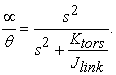(13)

Substituting for the known terms in eqs. (6), (7), (9) and (10), to obtain,(14)

Knowing that the tip deflection is,(15)

Hence, for any given value of, the tip deflection δ can be controlled by varying ϕ.

4. Results and Discussion

The results obtained from this investigation are presented in Figure 7 - Figure 12, showing the variation of tip deflection with time for the three cases of tip load, namely, no load, 27.4 gm and 59.5 gm. Two allowable tip deflection reductions were considered while activating the proposed control system, namely 50 % and 80 %. It can be observed that as the control system is activated, the frequency of oscillation of the beam is increased and the time required to reach the steady state condition is decreased.Download asVeiw figureFigures index
Figure 7. Tip deflection versus time, m payload = 0.0 gm, allowable tip deflection = 50%Download asVeiw figureFigures index
Figure 8. Tip deflection versus time, m payload = 0.0 gm, allowable tip deflection = 80%Download asVeiw figureFigures index
Figure 9. Tip deflection versus time, m payload = 27.4 gm, allowable tip deflection = 50%Download asVeiw figureFigures index
Figure 10. Tip deflection versus time, m payload = 27.4 gm, allowable tip deflection = 80%Download asVeiw figureFigures index
Figure 11. Tip deflection versus time, m payload = 59.5 gm, allowable tip deflection = 50%Download asVeiw figureFigures index
Figure 12. Tip deflection versus time, m payload = 59.5 gm, allowable tip deflection = 80%

The results of the frequency of oscillation and the time required to reach the steady state condition, are summarized and tabulated in Table 3 for all the cases studied.

Table 3. frequency and settling time for the cases studied

From the results obtained, it can be seen that as the percentage allowable reduction in tip deflection increases, the frequency of oscillation increases and the settling time decreases, this is obviously true due to the increase in the beam lateral stiffness.

5. Conclusions

In this work the control of tip deflection of a flexible link moving in a horizontal plane is addressed. The main targets of this investigation were to decrease the tip deflection together with decreasing the settling time, hence the steady state condition can be reached faster and the final position of the beam is achieved with minimal vibration. The adopted control procedure based on increasing the lateral stiffness proved its activity through the tests achieved in this study. The future work is aimed to extend the use of this method for a flexible beam moving in a 3-D space.

References

  V. Feliu, K.S. Rattan and H.B. Brown, Jr., Modeling and control of single-link flexible arms with lumped masses, Journal of dynamic systems, Measurement, and Control, ASME, Vol.114, 1992, PP. 59-69.In article  T. Singh, M.F. Golnaraghi and R.N. Dubey, Sliding – Mode / Shaped – Input Control of Flexible / Rigid Link Robots, Journal of Sound and Vibration, Vol. 171(2), 1994, PP. 185-200.In article  Mehrdad Moallem, Control and Design of Flexible- Link Manipulator, Ph.D. Thesis Concordia University Montreal, Quebec, Canada, 1996.In article  Jan-Peter Hauschild, Control of a Flexible Link Robotic Manipulator in zero gravity condition, A report presented to the University of Waterloo in fulfillment of the requirements for GENE 503, 2003.In article  Victor Etxebarria, Arantza Sanz and Ibone Lizarraga, Control of a light weight flexible robotic arm using sliding modes, International Journal of Advanced Robotic Systems, Vol. 2, 2005, PP.103-110.In article  Juan Fernando Peza Solis, Gerardo Silvanavarro and Rafael Castro Linares, Modeling and tip position control of a flexible link robot: Experimental results, Computation Systems, Vol.12, No.4, 2009, PP.421-435.In article  J.V.C. de Castro and L.C.G de Souza, Comparison of the LQG and H-Infinity Techniques to Design Experimentally a Flexible Satellite Attitude Control System, Journal of Aerospace Engineering, Sciences and Application, Vol. II, No.2, 2010, PP.17-25.In article  T. Mansour, A. Konno, and M. Uchiyama, Modified PID control of a single-link flexible robot, Advanced Robotics, Vol.22, Issue 4, pp. 433-449, 2010.In article  Jacob Katz and David W. Miller, Estimation and Control of Flexible Space Structures for Autonomous On-Orbit Assembly, M.Sc. thesis, Department of Aeronautics and Astronautics – Massachusetts Institute of Technology, June 2009.In article  Panos J. Antsaklis, Intelligent Control, Encyclopedia of Electrical and Electronics Engineering, Vol. 10, pp. 493-503, John Wiley & Sons, Inc., 1999.In article  Lind Zhixia Shi and Mohamed B. Trabia, Design and Tuning of Importance-based fuzzy logic controller for a flexible link manipulator, Journal of Intelligent and Fuzzy Systems, Vol.17, issue 3, PP.313-323, 2005.In article  J.M. Renno, Inverse dynamics-based tuning of a fuzzy logic controller for s single-link manipulator, Journal Vibration Control, Vol.13, 2007, PP. 1741-1759.In article  J. Shi, W. Zheng, J. Li and D. Chen, A distributed fuzzy logic controller based attitudinal control for single-link flexible manipulator,, IEEE Symposium of Electrical & Electronics Engineering, 2012, Kula Lampur, Malaysia, PP.357-359.In article  Ismail Hakki Akyuz, Zafer Bingul and Selcuk Kizir, Cascade fuzzy logic control of a single-link flexible –joint manipulator, Turkish Journal of Electrical Engineering & Computer Science, Vol.20, No.5, 2012, PP. 713-726.In article  Yuan-Gang Tang, Fu-Chun Sun, Zeng-Qi Sun and Ting-Liang Hu, Tip position control of a flexible-link manipulator with neural networks, International Journal of Control Automation and Systems, Vol.4, 2006, PP.308-317.In article  M. Tinkir, U. Onen and M. Kalyoncu, Modelling of neuro fuzzy control of a flexible link robot manipulator, Proceedings of the institution of Mechanical Engineers, Part I, System Control Engineering, Vol.224, 2010, PP.529-543.In article  A. Farmanbordar and S.M. Hoseini, Neural Network adaptive output feedback control of flexible link manipulators, Journal of Dynamic Systems Measurement and Control, Vol. 135, Issue 2, 2012.In article View Article  M.S. Alam and M.O. Tokhi, Designing feedforward command shapers with multi-objective genetic optimization for vibration control of a single-link flexible manipulator, Engineering Applications of Artificial Intelligence, Vol.21, Issue 7, pp. 229-246, 2008.In article View Article  Malik Loudini, Modelling and intelligent control of an elastic link robot manipulator, International Journal of Advances Robotic Systems, Vol. 10, Issue 1, 2013.In article View Article  S. Khajehpour and V. Azadi, Vibration suppression of a rotating flexible cantilever pipe conveying fluid using piezoelectric layers, Latin American Journal of Solids and Structures (lajss), Vol. 12, pp 1042-1060, 2015.In article View Article  Liang Zhao and Zhen-Dong Hu, Active vibration control of an axially translating robot arm with rotating prismatic joint using self-sensing actuator, Shock and Vibration, Hindawi Publishing Corporation, Vol. 2015, Article ID 964139.In article  K.B. Waghulde, Bimleshkumar Sinha, M.M. Patil and S. Mishra, Vibration control of cantilever smart beam by using piezoelectric actuators and sensors, International Journal of Engineering and Technology, Vol. 2, Issue 4, pp. 259-262, 2010.In article  Saurabh Kumar, Rajeev Srivastava and R.K. Srivastava, Active vibration control of smart piezo cantilever beam using PID controller, International Journal of Research in Engineering and Technology, Vol. 3, Issue 1, pp. 392-399, 2014.In article  Riessom Weldegiorgis, Prasad Krishna and K.V. Gangadharan, Vibration control of smart cantilever beam using strain rate feedback, International Conference on Advances in Manufacturing and Materials Engineering AMME 2014, Procedia Material Science 5(2014), pp. 113-122.In article